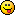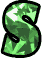# Math Is Fun Forum

Discussion about math, puzzles, games and fun.   Useful symbols: ÷ × ½ √ ∞ ≠ ≤ ≥ ≈ ⇒ ± ∈ Δ θ ∴ ∑ ∫  π  -¹ ² ³ °

You are not logged in.

## #1 2010-10-29 00:39:29

JaneFairfax
MemberRegistered: 2007-02-23
Posts: 6,868

### Homeomorphism of Euclidean plane to sphere with point removed

It is clear that
is not homeomorphic to a sphere (by which I mean the surface only, not the interior). The latter is compact whereas the former isnt. However if you remove a single point from the sphere, then the two spaces are homeomorphic.

Let us take the unit sphere centred at

, given by
, and let us remove the north pole
. Call this pointless sphere
. Then
is the disjoint union of circles formed by the intersection of
with the plane
as t varies from 0 to 2 (including 0 but not 2).

Now consider

itself. This is the disjoint union of origin-centred circles of all possible non-negative radii (counting
as a circle of radius 0). Let
be any continuous bijection (e.g.
or
).

Then if we define

by
and for

[align=center]

[/align]
where
, we have a homeomorphism!

I was thinking about this last night. Thinking about math problems is a great way to pass the time when youre having insomnia.Last edited by JaneFairfax (2010-10-29 01:50:05)

Offline

## #2 2011-01-14 23:58:13

scientia
MemberRegistered: 2009-11-13
Posts: 224

### Re: Homeomorphism of Euclidean plane to sphere with point removed

The surface of the sphere is homeomorphic to the extended complex plane
.

Offline

## #3 2011-01-15 07:58:17

coprime
Member
Registered: 2011-01-15
Posts: 1

### Re: Homeomorphism of Euclidean plane to sphere with point removed

since homeomorphism is defined by continuity but does not require smoothness we can map the punctured sphere to sharpened pencil shape: a truncated half-cone with apex at the south pole intersecting the sphere at the equator, glued to a half infinite cylinder. then the cylinder is easily mapped to the remaining (infinite) portion of the cone. finally flatten out the cone.

use the unit sphere centred at the origin:

for the map of lower hemisphere to truncated half-cone we send
(x,y,z) to (px,py,z) where p=√ (1-z²)

for the map of the upper punctuated hemisphere to the half- cylinder we send
(x,y,z) to (x/r,y/r,zq/r) where r=√ (x²+y²) and q=√(1-r²)

to map the half-cyclinder to the remainder of the half-cone
send (x,y,z) to (-ipx,-ipy,z) where i=√(-1)

to flatten the half-cone send (x,y,z) to (x,y)

(NB the use of imaginaries is merely a notational convenience)

Last edited by coprime (2011-01-15 08:14:11)

Offline